www.batmath.it

### The integral of step functions

Consider a step function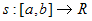and a dissectionof [a,b] such that the function has the constant value ck on each subinterval of the dissection. Then:

The integral of s from a to b is the number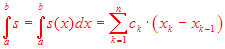.

This means that the integral is simply the sum of the products of the constants ck times the length of the corresponding subintervals. In particular if f is constant and positive the integral is the area of a rectangle.

The two symbolsandare used without distinction: we prefer the first, or sometimes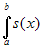, because it is more compact, but the second one has some advantages, in particular when we deal with multi-variable functions or in the use of the substitution rule. It is important, at any rate, to remember that the integral depends only on the function s and the interval [a,b]: the symbol "dx" has no particular meaning and is not a differential.

#### Examples

• Consider the function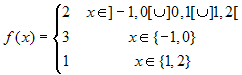. Then the integral from -1 to 2 is the area of the rectangle ABCD in the picture here below.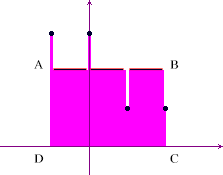• Consider the function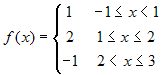. Then the integral from -1 to 3 is the sum of the areas of the two yellow rectangles minus the area of the green rectangle.The two examples above prove that the integral of a step function is the algebraic sum of the areas of some rectangles: rectangles above the x-axis are taken with a positive area, those below the x-axis are taken with negative area.

Observe in particular that the values of the step functions at the points of the dissection are unimportant: these numbers are never used in the previous definition. This means that if you modify the values of a step function in a finite number of points (obviously changing also the dissection of the interval [a,b]) the value of the integral is not affected. This agrees with the fact that the area of a segment is zero. From now on we'll no more graph these points.

It's also important to observe that continuity of the functions is not important for the concept of integral: step functions are never continuous (except when constant!).

In the above definition of integral the number a is less than b. This definition is usually extended, in order to allow also a>b, as follows: if a>b then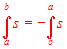. We also define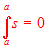.

first published on january 07 2003 - last updated on september 01 2003